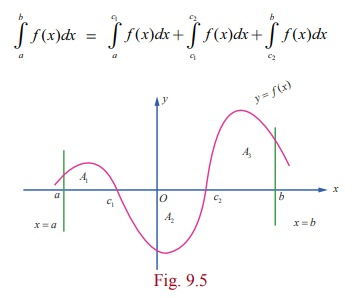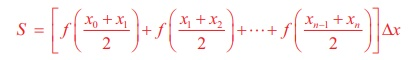Home | | Maths 12th Std | Definite Integral as the Limit of a Sum

# Definite Integral as the Limit of a Sum

Consider a real-valued, bounded function f (x) defined on the closed and bounded interval[a, b ], a < b.

Definite Integral as the Limit of a Sum

Riemann Integral

Consider a real-valued, bounded function f (x) defined on the closed and bounded interval[a, b ], a < b. The function f ( x) need not have the same sign on [a , b] ; that is, f ( x) may have positive as well as negative values on [a , b] . See Fig 9.2. Partition the interval [a , b] into n subintervals [x0 , x1 ],[x1 , x2 ], ŌĆ” ,[xn ŌłÆ 2 , xnŌłÆ1 ],[xn ŌłÆ1 , xn ] such that

a = x0 < x1 < x2 < < xn ŌłÆ1 < xn = b.In each subinterval [xi ŌłÆ1 , xi ], i = 1, 2, ŌĆ”. , n, choose a real number ╬Ši arbitrarily such that xi ŌłÆ1 Ōēż ╬Ši Ōēż xi.

Consider the sum Ōłæni=1 f (╬Ši )( xi ŌłÆ xi ŌłÆ1 ) = f (╬Š1 )(x1 ŌłÆ x0 ) + f (╬Š2 )(x2 ŌłÆ x1 ) + ŌĆ” + f (╬Šn )(xn ŌłÆ xnŌłÆ1 ) ŌĆ”.(1)

The sum in (1) is called a Riemann sum of f ( x) corresponding to the partition [x0 , x1 ],[x1 , x2 ], . . . ,[xn ŌłÆ1 , xn ] of [a , b]. Since there are infinitely many values ╬Ši satisfying the condition xi ŌłÆ1 Ōēż ╬Ši Ōēż xi , there are infinitely many Riemann sums of f ( x) corresponding to the same partition [x0 , x1 ],[x1 , x2 ], . . . ,[xn ŌłÆ1 , xn ] of [a , b]. If, under the limiting process n ŌåÆ Ōł× and max ( xi ŌłÆ xi ŌłÆ1)ŌåÆ 0, the sum in (1) tends to a finite value, say A, then the value A is called the definite integral of f ( x) with respect to x on [a , b] . It is also called the Riemann integral of f ( x) on [a , b] and is denoted by bŌł½a f ( x)dx and is read as the integral of f ( x) with respect to x from a to b . If a = b, then we have aŌł½a f ( x)dx = 0.

Note

In the present chapter, we consider bounded functions f ( x) that are continuous on[a , b] . However, the Riemann integral of f ( x) on [a , b] also exists for bounded functions f ( x) that are piece-wise continuous on[a , b] .We have used the same symbol Ōł½ both for definite integral and anti-derivative (indefinite integral). The reason will be clear after we state the Fundamental Theorems of Integral Calculus. The variable x is dummy in the sense that it is selected at our choice only. So we can write bŌł½a f (x)dx as bŌł½a f (u)du . So, we have bŌł½a f (x)dx = bŌł½a f (u)du . As max ( xi ŌłÆ xiŌłÆ1) ŌåÆ 0, all the three points xi ŌłÆ1 , ╬Ši , and xi of each subinterval [ xi ŌłÆ1 , xi ] are dragged into a single point. We have already indicated that there are infinitely many ways of choosing the evaluation point ╬Ši in the subinterval [ xi ŌłÆ1 , xi ] , i = 1, 2, . . . , n . By choosing ╬Ši =xi ŌłÆ1 , i = 1, 2, , n , we haveEquation (2) is known as the left-end rule for evaluating the Riemann integral.

By choosing ╬Ši =xi , i = 1, 2,. . . , n , we haveEquation (3) is known as the right-end rule for evaluating the Riemann integral.Equation (4) is known as the mid-point rule for evaluating the Riemann integral.

Remarks

(1) If the Riemann integral bŌł½a f ( x)dx exists, then the Riemann integral xŌł½a f (u)du is a

well-defined real number for every x Ōłł[a, b] . So, we can define a function F ( x) on [a , b] such that F ( x) = xŌł½a f (u)du, x Ōłł[a, b] .

(2) If f (x) Ōēź 0 for all x Ōłł[a, b] , then the Riemann integral bŌł½af ( x)dx is equal to the geometric area of the region bounded by the graph of y = f ( x) , the x-axis, the lines x = a and x = b . See Fig. 9.3.(3) If f ( x) Ōēż 0 for all x Ōłł[a, b] , then the Riemann integral bŌł½a f ( x)dx is equal to the negative of the geometric area of the region bounded by the graph of y = f ( x) , the x-axis, the lines x = a and x = b . See Fig. 9.4. In this case, the geometric area of the region bounded by the graph of y = f ( x) , the x-axis, the lines x = a and x = b is given by(4) If f (x) takes positive as well as negative values on [a , b] , then the interval [a , b] can be divided into subintervals [a , c1 ] , [c1 , c2 ] ,. . .  , [ck , b] such that f (x) has the same sign throughout each of subintervals. So, the Riemann integral bŌł½a f (x)dx is given byIn this case, the geometric area of the region bounded by the graph of y = f ( x) , the x-axis, the lines x = a and x = b is given byFor instance, consider the following graph of a function f ( x), x Ōłł[a, b] . See Fig. 9.5. Here, A1 , A2 and, A3 denote geometric areas of the individual parts.

Then, the definite integral bŌł½a f ( x)dx is given by= A1ŌłÆA2+A3.

The geometric area of the region bounded by the graph of y = f ( x) , the x ŌłÆ axis, the lines x = a and x = b is given by A1 + A2 + A3 . In view of the above discussion, it is clear that a Riemann integral need not represent geometrical area.

Note

Even if we do not mention explicitly, it is always understood that the areas are measured in square units and volumes are measured in cubic units.

Example 9.1

Estimate the value of Ōł½00.5 x2dx using the Riemann sums corresponding to 5 subintervals of equal width and applying (i) left-end rule (ii) right-end rule (iii) the mid-point rule.

Solution

Here a = 0, b = 0.5, n = 5, f (x) = x2

So, the width of each subinterval is

h = ╬öx = bŌłÆa / n  = 0.5ŌłÆ0 / 5 = 0.1.

The partition of the interval is given by the points

x0 = 0,

x1 = x0 + h = 0 + 0.1 = 0.1

x2 = x1 + h = 0.1+ 0.1 = 0.2

x3= x2 + h = 0.2 + 0.1 = 0.3

x4= x3 + h = 0.3 + 0.1 = 0.4

x5= x4 + h = 0.4 + 0.1 = 0.5

(i) The left-end rule for Riemann sum with equal width ╬öx is

S = [ f(x0) + f (x1) + . . . + f ( x nŌłÆ1 )╬öx .

S = [f ( 0) + f ( 0.1) + f ( 0.2) + f ( 0.3) + f ( 0.4) ] (0.1)

= [ 0.00 + 0.01+ 0.04 + 0.09 + 0.16] (0.1) = 0.03

Ōł┤ Ōł½00.5 x2 dx is approximately 0.03 .

(ii) The right-end rule for Riemann sum with equal width Dx is

S = [ f(x1) + f (x2) + . . . + f ( x n )╬öx .

S = [ f ( 0.1) + f ( 0.2) + f ( 0.3) + f ( 0.4) + f ( 0.5) ] (0.1)

= [ 0.01+ 0.04 + 0.09 + 0.16 + 0.25](0.1) = 0.055 .

Ōł┤ Ōł½00.5 x2 dx is approximately 0.055 .

(iii) The mid-point rule for Riemann sum with equal width ╬öx isS = [ f ( 0.05) + f ( 0.15) + f ( 0.25) + f ( 0.35) + f ( 0.45) ] (0.1)

= [ 0.0025 + 0.0225 + 0.0625 + 0.1225 + 0.2025](0.1)

= 0.04125 .

Ōł┤ Ōł½00.5 x2dx is approximately 0.04125 .

Tags : Applications of Integration | Mathematics , 12th Maths : UNIT 9 : Applications of Integration
Study Material, Lecturing Notes, Assignment, Reference, Wiki description explanation, brief detail
12th Maths : UNIT 9 : Applications of Integration : Definite Integral as the Limit of a Sum | Applications of Integration | Mathematics